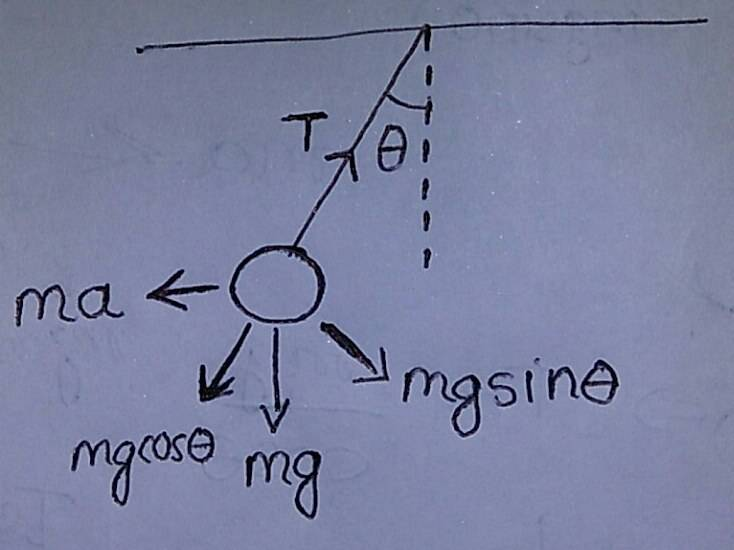# Pendulum Question

## Homework Statement

A pendulum is hanging from the ceiling of a cage. If the cage moves up with constant acceleration a, its tension is T1. If it moves down with the same acceleration a then the tension is T2. If the cage moves horizontally with the same acceleration a, then the tension is T. Now, 2T2 =
(a) T12 + T22
(b) T12 - T22
(c) 2T12 - T22
(d) T12 - 2T22

F = ma

## The Attempt at a Solution

I found the tension in the string for the above two cases from their respective Free Body Diagrams
i.e T1 = m(g+a) and T2 = m(g-a)
But I had some problems for the Free Body Diagram of the pendulum in the third case, i.e when the cage is accelerating sideways.
Here is my FBDSo from the FBD I get
T = mg cos θ ---(i) and tan θ = (a/g) ---(ii)
My confusion is that is the equation (i) correct ?
Since the equations in the book is given in the book is written differently
Instead of resolving the weight components they resolved the tension components i.e the equations are given as
T sin θ = ma --- (iii)
T cos θ = mg ---(iv)
As you can see both the equations (i) and (iv) looks different. I could not figure out where is the mistake

## Answers and Replies

Orodruin
Staff Emeritus
Science Advisor
Homework Helper
Gold Member
There are three forces acting on the pendulum. You are confusing yourself by introducing the components only of the gravitational force. Start by drawing the FBD without a coordinate system, then introduce a coordinate system and write down the force equations in all directions.

•Aninda
Orodruin
Staff Emeritus
Science Advisor
Homework Helper
Gold Member
Alternatively, you can just use the fact that the net force needs to be zero and that a and g are orthogonal ... Then you never need to introduce a coordinate system or care about the components.

•Aninda
There's no mistake, he just broke down the tension in x and y-direction. Try to draw a graph with two axes (x, y) and draw on it the decomposed tension in x and y-direction, the cage force (ma) and the weight force (mg), and you will see what the book did. I hope that someone more qualified helps you, but for now, think about it.
(Sorry, the message came too late, someone has already answered the question)